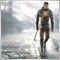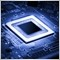• Overview
• Reviews (5)
• What's new

For opening positions, the Expert Advisor uses signals from the Relative Strength Index (RSI) indicator.

Input Parameters:

• Period of RSI - RSI period;
• Signal level in percents - level the line should cross to generate a signal for opening a position;
• Take in atr, 0 - do not use a take profit - take profit size calculated using ATR (50) applied to the current timeframe. If the parameter value is 0, it is not used;
• Stop in atr, 0 - do not use a stop - stop loss size. If the parameter value is 0, it is not used;
• Trailing in atr, 0 - do not use a trailing - trailing stop size. If the parameter value is 0, it is not used;
• Risk factor, volume eq Equty*Risk factor/1000 - used in the work lot calculation formula: Equity*Risk factor/1000. Suppose, Equity is 10000 and Risk factor is 0.01, then the volume is 10000*0.01/1000 = 0.1;
• Volume in lots if Risk factor is 0 - work lot size. If you set the Risk factor to 0, the specified fixed lot will be used.

The RSI indicator is an oscillator, thus it is recommended to use it on the instruments with rather less trend periods. Price movements caught are also small, thus it is recommended to use close take profits and stop losses.

The screenshots demonstrate the results of backtesting on EURGBP. The test was conducted for the period from 2003.05.13 to 2013.05.13 on D1 timeframe.16098
Nikolay Gaylis 2019.01.14 06:46

User didn't leave any comment to the rating7622
2017.10.18 08:35

User didn't leave any comment to the rating7182
2016.01.24 00:19

User didn't leave any comment to the rating1440